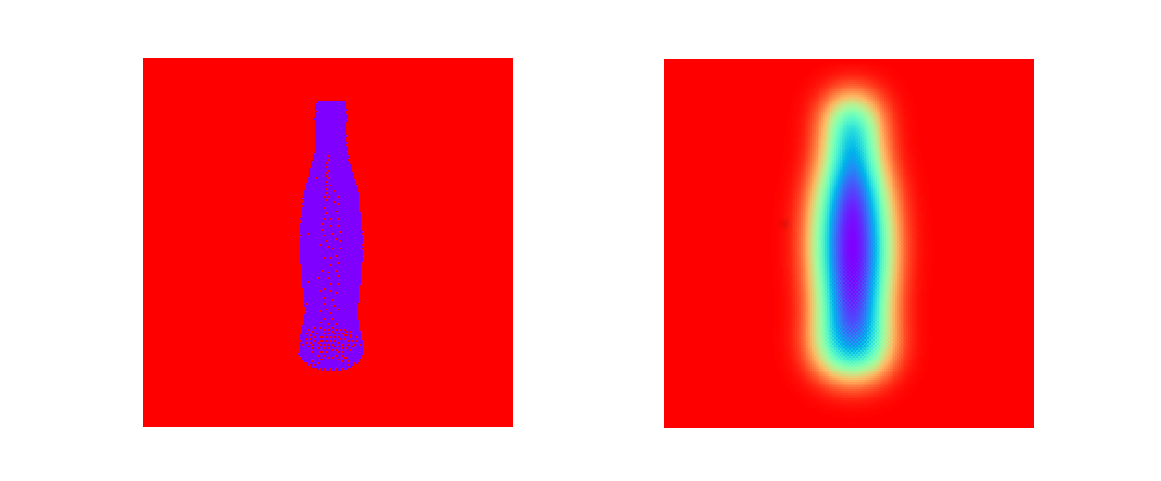1.12

# Introducing heat equation

Heat (or diffusion) equation is a partial differential equation that describes how the temperature varies in space over time.

The numerical solution of the heat equation contains performance aspects that are present also in many other problems and, as such, the heat equation is used as an example in several hands-on exercises throughout the course.

The heat equation can be written as

where u(x, y, t) is the temperature field that varies in space and time, and α is the thermal diffusivity constant.

The equation can be solved numerically in two dimensions by discretizing first the Laplacian with finite differences as

Given an initial condition ($u(t=0) = u_0$) one can then follow the time dependence of the temperature field with the explicit time evolution method:

Note: the algorithm is stable only when

The image below shows a result of numerical simulation where the initial state is a cold object in a hot surrounding (for us Finns this would be a soda bottle in a sauna) and we follow the time evolution for few hundres of time steps.Are you familiar with any other problems with a similar numerical solution? Please comment!